# High performance algorithms based on a new wavelet expansion for time dependent acoustic obstacle scattering*

## Abstract

We present a highly parallelizable numerical method to solve time dependent acoustic obstacle scattering problems. The method proposed is a generalization of the "operator expansion method" developed in . Using a Fourier transform with respect to time the time dependent scattering problem for the wave equation considered is transformed in an exterior boundary value problem for the Helmholtz equation depending on a parameter. The "operator expansion method" is used to solve the exterior problems for the Helmholtz equation and reduces, via a perturbative approach, the solution of each exterior problem to the solution of a sequence of systems of first kind integral equations defined on a suitable "reference surface". The numerical solution of these systems of integral equations is challenging when scattering problems involving realistic obstacles and wavelengths small compared to the characteristic dimensions of the obstacles are solved. Let RT be the ratio between the characteristic dimension of the obstacle and the wavelength considered. In the numerical experiments presented the greater ratios RT considered range in the interval [13,60]. This implies the use of high dimensional vector spaces to approximate satisfactorily the corresponding systems of integral equations. That is each system of integral equations in the sequence mentioned above after being discretized to be solved numerically becomes a large system of linear equations. Represented on a generic base the matrices that approximate the integral operators after the discretization will be dense matrices. We introduce a new way of using the wavelet transform and new bases of wavelets and we develop a version of the operator expansion method that constructs directly element by element the coefficient matrix of the sparse linear systems that approximate in the wavelet basis considered the systems of integral equations. The resulting numerical method, using affordable computing resources, is able to deal with realistic acoustic scattering problems solving large (sparse) linear systems (up to approximately 5. 105 (real) unknowns and equations in the numerical experience shown here). The computation of the elements of the coefficient matrices of the linear systems considered and several other aspects of the numerical algorithm proposed are highly parallelizable allowing the development of an efficient solver for exterior boundary value problems for the Helmholtz equation. Several numerical experiments involving realistic obstacles and "small" wavelengths are proposed. When possible the quantitative character of the numerical results obtained is established. To evaluate the performance of the proposed algorithm on parallel computing facilities appropriate speed up factors are introduced and evaluated. Some animations and virtual reality applications relative to these numerical experiments can be found in this website.

## References


M. C. Recchioni and F. Zirilli, The use of wavelets in the operator expansion method for time dependent acoustic obstacle scattering, SIAM J. Sci. Comput., 25 (2003), pp. 1158-1186.

* The numerical experience reported in this website has been obtained using the computing grid of Enea (Roma, Italy). The support and sponsorship of Enea (Roma, Italy) is gratefully acknowledged.

Warning: To see the virtual reality applications we recommend to use at least the following resources:
```- screen resolution: 1024 x 768
- processor: Pentium II or equivalent
```

We suggest the following VRML clients:

 Windows Mac UNIX/Linux Cortona X X Cosmo Player X X OpenVRML (Under Developement) X FreeWRL (Under Developement) X

Note that in a Unix/Linux environment the clients OpenVRML and FreeWRL allow the visualization of virtual objects but not the visualization of virtual scenes.

Time harmonic scattering from an acoustically soft sphere

Data: incident wave: ui=exp(-i(t-z)), c=1, radius of the sphere r  =1, RT=1/p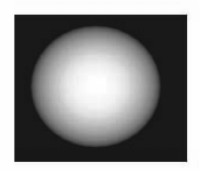Sphere (virtual object): VRML visualization

The following table shows the comparison between the approximate solution obtained using the method proposed here and the approximate solution obtained truncating at l=Lmax=10 the spherical harmonics expansion of the solution.

Time dependent scattering from a simplified version of a US Navy submarine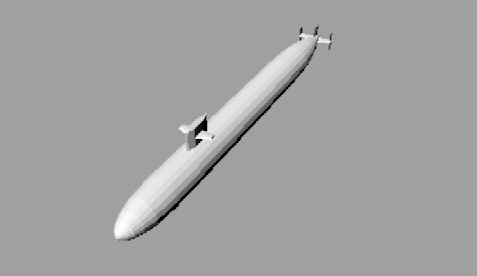Original submarine: VRML visualization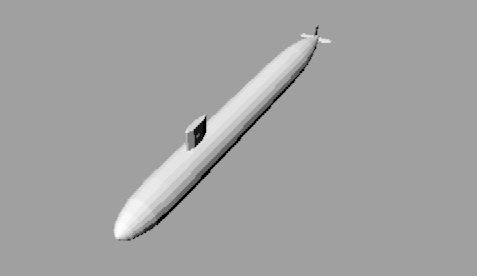Simplified Version of the submarine: VRML visualization

Remark. In the following animations the time values shown in the twenty frames are t=-70+(140/c)j/19, j=0,1,...,19, c=1532.8 meters/seconds.

Time depedent scattering from the simplified version of the submarine: Data: incident wave ui=exp(-4(z+ct)2), c=1532.8 meters/seconds (sound speed in the sea water), length of the simplified version of the submarine L=110.3   meters, 1.5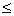RT13 Animation 1: Click here to see the scattering phenomenon (Virtual scene: VRML file)

Time harmonic scattering from a simplified version of the NASA space shuttleNASA shuttle: VRML visualization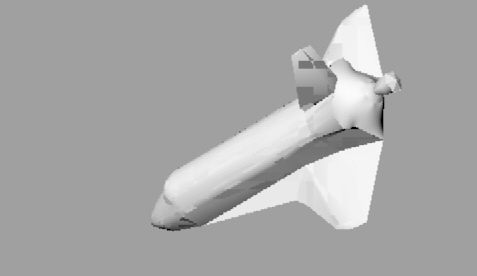Simplified Version of the NASA space shuttle: VRML visualization

Remark. In the following animations the time values shown in the twenty frames are t=(2p/w) j/19, j=0,1,...,19,

w=(2p/3)c, c=331.45 meters/seconds

Time harmonic scattering from the simplified version of the Nasa space shuttle

Data: incident wave ui=exp(-i2p(z+ct)/3), c=331.45 meters/seconds (sound speed in the air), length of the simplified version of the NASA space shuttle L=56.14   meters, RT=14/3

Animation 2: Click here to see the scattering phenomenon (Virtual scene: VRML file)

The following figure shows the time harmonic scattering from the simplified version of Nasa space shuttle when the incident wave is ui=exp(-i8p(z+ct)) and c=331.45 meters/seconds, this corresponds to RT=56. Note that Re(*) means real part of the complex number * .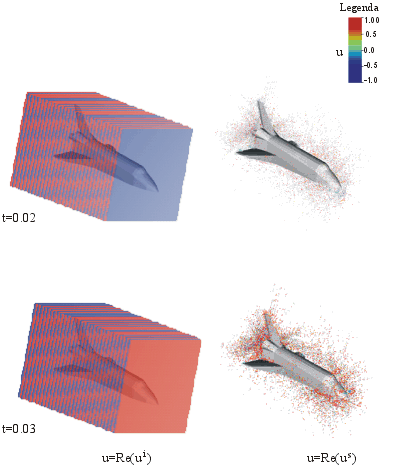Entry n.